# ScapeGoat Tree | Set 1 (Introduction and Insertion)

• Difficulty Level : Hard
• Last Updated : 07 Jul, 2022

A ScapeGoat tree is a self-balancing Binary Search Tree like AVL Tree, Red-Black Tree, Splay Tree, ..etc.

• Search time is O(Log n) in worst case. Time taken by deletion and insertion is amortized O(Log n)
• The balancing idea is to make sure that nodes are α size balanced. Α size balanced means sizes of left and right subtrees are at most α * (Size of node). The idea is based on the fact that if a node is Α weight balanced, then it is also height balanced: height <= log1/&aplpha;(size) + 1
• Unlike other self-balancing BSTs, ScapeGoat tree doesn’t require extra space per node. For example, Red Black Tree nodes are required to have color. In below implementation of ScapeGoat Tree, we only have left, right and parent pointers in Node class. Use of parent is done for simplicity of implementation and can be avoided.

Insertion (Assuming α = 2/3): To insert value x in a Scapegoat Tree:

• Create a new node u and insert x using the BST insert algorithm.
• If the depth of u is greater than log3/2n where n is number of nodes in tree then we need to make tree balanced. To make balanced, we use below step to find a scapegoat.
• Walk up from u until we reach a node w with size(w) > (2/3)*size(w.parent). This node is scapegoat
• Rebuild the subtree rooted at w.parent.

What does rebuilding the subtree mean?
In rebuilding, we simply convert the subtree to the most possible balanced BST. We first store inorder traversal of BST in an array, then we build a new BST from array by recursively dividing it into two halves.

```        60                            50
/                           /     \
40                          42       58
\          Rebuild      /    \    /   \
50       --------->  40      47 55    60
\
55
/   \
47     58
/
42 ```

Below is C++ implementation of insert operation on Scapegoat Tree.

## C++

 `// C++ program to implement insertion in``// ScapeGoat Tree``#include``using` `namespace` `std;` `// Utility function to get value of log32(n)``static` `int` `const` `log32(``int` `n)``{``  ``double` `const` `log23 = 2.4663034623764317;``  ``return` `(``int``)``ceil``(log23 * ``log``(n));``}` `// A ScapeGoat Tree node``class` `Node``{``public``:``  ``Node *left, *right, *parent;``  ``float` `value;``  ``Node()``  ``{``    ``value = 0;``    ``left = right = parent = NULL;``  ``}``  ``Node (``float` `v)``  ``{``    ``value = v;``    ``left = right = parent = NULL;``  ``}``};` `// This functions stores inorder traversal``// of tree rooted with ptr in an array arr[]``int` `storeInArray(Node *ptr, Node *arr[], ``int` `i)``{``  ``if` `(ptr == NULL)``    ``return` `i;` `  ``i = storeInArray(ptr->left, arr, i);``  ``arr[i++] = ptr;``  ``return` `storeInArray(ptr->right, arr, i);``}` `// Class to represent a ScapeGoat Tree``class` `SGTree``{``private``:``  ``Node *root;``  ``int` `n; ``// Number of nodes in Tree``public``:``  ``void` `preorder(Node *);``  ``int` `size(Node *);``  ``bool` `insert(``float` `x);``  ``void` `rebuildTree(Node *u);``  ``SGTree()   { root = NULL; n = 0; }``  ``void` `preorder() { preorder(root); }` `  ``// Function to built tree with balanced nodes``  ``Node *buildBalancedFromArray(Node **a, ``int` `i, ``int` `n);` `  ``// Height at which element is to be added``  ``int` `BSTInsertAndFindDepth(Node *u);``};` `// Preorder traversal of the tree``void` `SGTree::preorder(Node *node)``{``  ``if` `(node != NULL)``  ``{``    ``cout << node->value << ``" "``;``    ``preorder(node -> left);``    ``preorder(node -> right);``  ``}``}` `// To count number of nodes in the tree``int` `SGTree::size(Node *node)``{``  ``if` `(node == NULL)``    ``return` `0;``  ``return` `1 + size(node->left) + size(node->right);``}` `// To insert new element in the tree``bool` `SGTree::insert(``float` `x)``{``  ``// Create a new node``  ``Node *node = ``new` `Node(x);` `  ``// Perform BST insertion and find depth of``  ``// the inserted node.``  ``int` `h = BSTInsertAndFindDepth(node);` `  ``// If tree becomes unbalanced``  ``if` `(h > log32(n))``  ``{``    ``// Find Scapegoat``    ``Node *p = node->parent;``    ``while` `(3*size(p) <= 2*size(p->parent))``      ``p = p->parent;` `    ``// Rebuild tree rooted under scapegoat``    ``rebuildTree(p->parent);``  ``}` `  ``return` `h >= 0;``}` `// Function to rebuilt tree from new node. This``// function basically uses storeInArray() to``// first store inorder traversal of BST rooted``// with u in an array.``// Then it converts array to the most possible``// balanced BST using buildBalancedFromArray()``void` `SGTree::rebuildTree(Node *u)``{``  ``int` `n = size(u);``  ``Node *p = u->parent;``  ``Node **a = ``new` `Node* [n];``  ``storeInArray(u, a, 0);``  ``if` `(p == NULL)``  ``{``    ``root = buildBalancedFromArray(a, 0, n);``    ``root->parent = NULL;``  ``}``  ``else` `if` `(p->right == u)``  ``{``    ``p->right = buildBalancedFromArray(a, 0, n);``    ``p->right->parent = p;``  ``}``  ``else``  ``{``    ``p->left = buildBalancedFromArray(a, 0, n);``    ``p->left->parent = p;``  ``}``}` `// Function to built tree with balanced nodes``Node * SGTree::buildBalancedFromArray(Node **a,``                ``int` `i, ``int` `n)``{``  ``if` `(n== 0)``    ``return` `NULL;``  ``int` `m = n / 2;` `  ``// Now a[m] becomes the root of the new``  ``// subtree a,.....,a[m-1]``  ``a[i+m]->left = buildBalancedFromArray(a, i, m);` `  ``// elements a,...a[m-1] gets stored``  ``// in the left subtree``  ``if` `(a[i+m]->left != NULL)``    ``a[i+m]->left->parent = a[i+m];` `  ``// elements a[m+1],....a[n-1] gets stored``  ``// in the right subtree``  ``a[i+m]->right =``    ``buildBalancedFromArray(a, i+m+1, n-m-1);``  ``if` `(a[i+m]->right != NULL)``    ``a[i+m]->right->parent = a[i+m];` `  ``return` `a[i+m];``}` `// Performs standard BST insert and returns``// depth of the inserted node.``int` `SGTree::BSTInsertAndFindDepth(Node *u)``{``  ``// If tree is empty``  ``Node *w = root;``  ``if` `(w == NULL)``  ``{``    ``root = u;``    ``n++;``    ``return` `0;``  ``}` `  ``// While the node is not inserted``  ``// or a node with same key exists.``  ``bool` `done = ``false``;``  ``int` `d = 0;``  ``do``  ``{``    ``if` `(u->value < w->value)``    ``{``      ``if` `(w->left == NULL)``      ``{``        ``w->left = u;``        ``u->parent = w;``        ``done = ``true``;``      ``}``      ``else``        ``w = w->left;``    ``}``    ``else` `if` `(u->value > w->value)``    ``{``      ``if` `(w->right == NULL)``      ``{``        ``w->right = u;``        ``u->parent = w;``        ``done = ``true``;``      ``}``      ``else``        ``w = w->right;``    ``}``    ``else``      ``return` `-1;``    ``d++;``  ``}``  ``while` `(!done);` `  ``n++;``  ``return` `d;``}` `// Driver code``int` `main()``{``  ``SGTree sgt;``  ``sgt.insert(7);``  ``sgt.insert(6);``  ``sgt.insert(3);``  ``sgt.insert(1);``  ``sgt.insert(0);``  ``sgt.insert(8);``  ``sgt.insert(9);``  ``sgt.insert(4);``  ``sgt.insert(5);``  ``sgt.insert(2);``  ``sgt.insert(3.5);``  ``printf``(``"Preorder traversal of the"``    ``" constructed ScapeGoat tree is \n"``);``  ``sgt.preorder();``  ``return` `0;``}`

Output

```Preorder traversal of the constructed ScapeGoat tree is
7 6 3 1 0 2 4 3.5 5 8 9 ```

Example to illustrate insertion:

A scapegoat tree with 10 nodes and height 5.

```
7
/  \
6    8
/      \
5        9
/
2
/  \
1    4
/    /
0    3

Let’s insert 3.5 in the below scapegoat tree.```

Initially d = 5 < log3/2n where n = 10;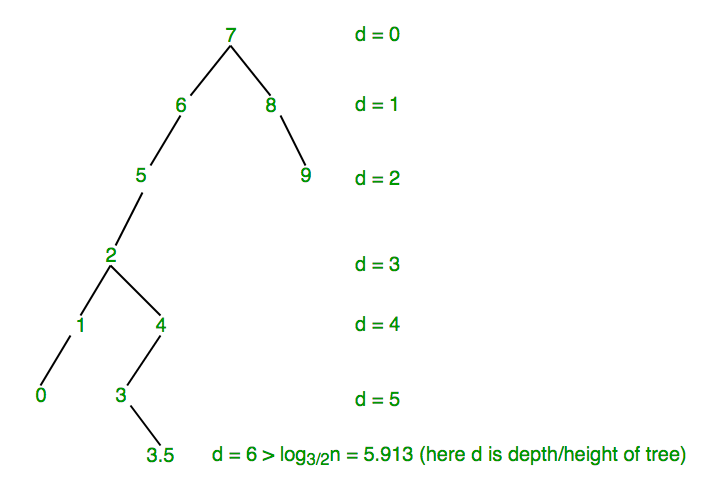Since, d > log3/2n i.e., 6 > log3/2n, so we have to find the scapegoat in order to solve the problem of exceeding height.

• Now we find a ScapeGoat. We start with newly added node 3.5 and check whether size(3.5)/size(3) >2/3.
• Since, size(3.5) = 1 and size(3) = 2, so size(3.5)/size(3) = ½ which is less than 2/3. So, this is not the scapegoat and we move up .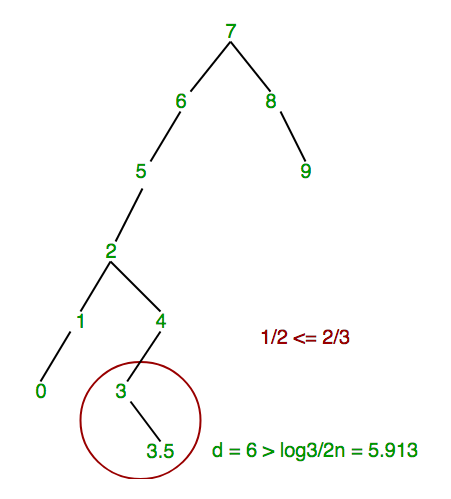• Since 3 is not the scapegoat, we move and check the same condition for node 4. Since size(3) = 2 and size(4) = 3, so size(3)/size(4) = 2/3 which is not greater than 2/3. So, this is not the scapegoat and we move up .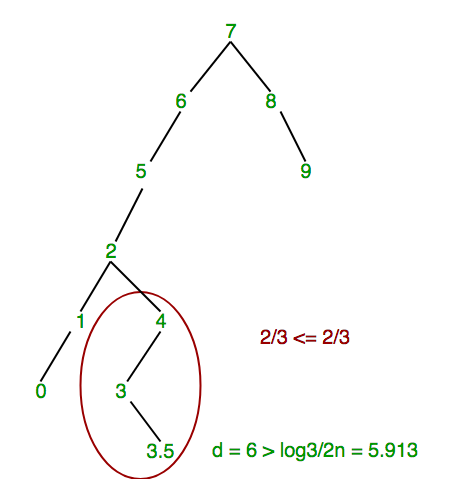• Since 3 is not the scapegoat, we move and check the same condition for node 4. Since, size(3) = 2 and size(4) = 3, so size(3)/size(4) = 2/3 which is not greater than 2/3. So, this is not the scapegoat and we move up .
• Now, size(4)/size(2) = 3/6. Since, size(4)= 3 and size(2) = 6 but 3/6 is still less than 2/3, which does not fulfill the condition of scapegoat so we again move up.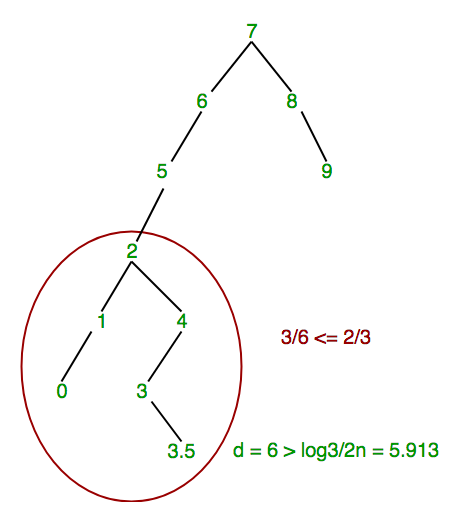• Now, size(2)/size(5) = 6/7. Since, size(2) = 6 and size(5) = 7. 6/7 >2/3 which fulfills the condition of scapegoat, so we stop here and hence node 5 is a scapegoat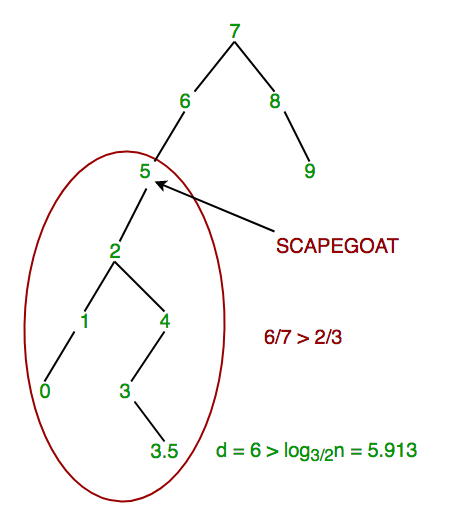Finally, after finding the scapegoat, rebuilding will be taken at the subtree rooted at scapegoat i.e., at 5. Final tree:

Comparison with other self-balancing BSTs

Red-Black and AVL : Time complexity of search, insert and delete is O(Log n)

Splay Tree : Worst case time complexities of search, insert and delete is O(n). But amortized time complexity of these operations is O(Log n).

ScapeGoat Tree: Like Splay Tree, it is easy to implement and has worst case time complexity of search as O(Log n). Worst case and amortized time complexities of insert and delete are same as Splay Tree for Scapegoat tree.

This article is contributed by Rahul Aggarwal and Sahil Chhabra (akku). If you like GeeksforGeeks and would like to contribute, you can also write an article and mail your article to review-team@geeksforgeeks.org. See your article appearing on the GeeksforGeeks main page and help other Geeks.

My Personal Notes arrow_drop_up